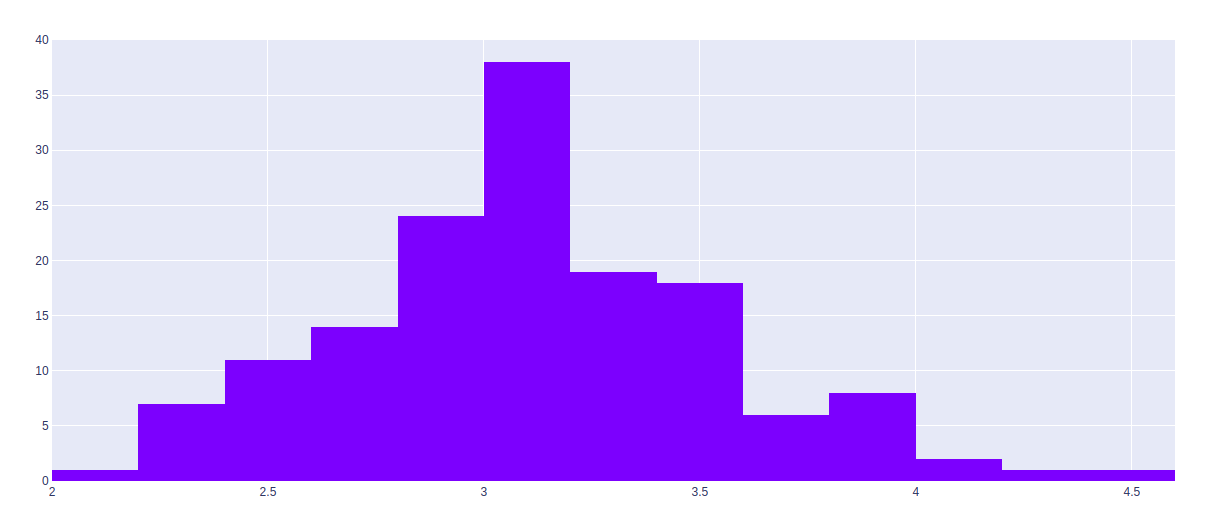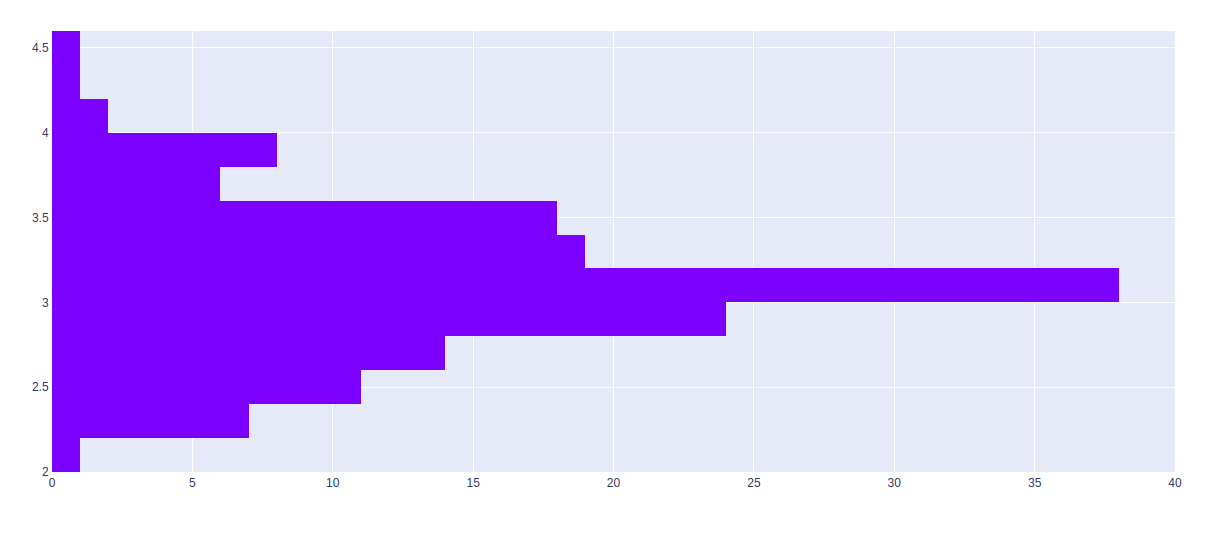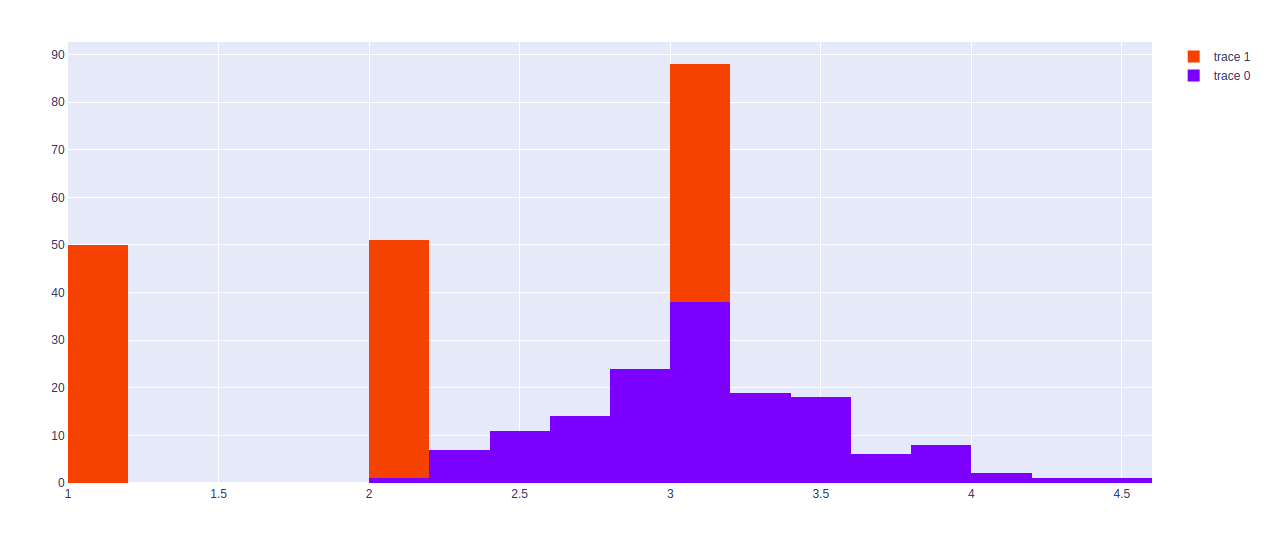# Histograms in Plotly using graph_objects class

Plotly is a Python library which is used to design graphs, especially interactive graphs. It can plot various graphs and charts like histogram, barplot, boxplot, spreadplot and many more. It is mainly used in data analysis as well as financial analysis. plotly is an interactive visualization library.

## Histogram using graph_objects class

Plotly provides the more generic go.Histogram class from plotly.graph_objects. But let us first understand what are hostograms. Histogram have few important parts, which is described below:

• The title: The title play a role to describes the information admitted in the histogram.
• X-axis: The X-axis are intervals that show the scale of values which measures all fall under.
• Y-axis: The Y-axis shows the number of times that the values occurred within the intervals set by the X-axis.
• The bars: The height of the bar shows the number of times that the values occurred within the interval, while the width of the bar shows the interval that is covered. For a histogram with equal bins, the width should be the same across all bars.

Syntax: class plotly.graph_objects.Histogram(arg=None, alignmentgroup=None, autobinx=None, autobiny=None, bingroup=None, cumulative=None, customdata=None, customdatasrc=None, error_x=None, error_y=None, histfunc=None, histnorm=None, hoverinfo=None, hoverinfosrc=None, hoverlabel=None, hovertemplate=None, hovertemplatesrc=None, hovertext=None, hovertextsrc=None, ids=None, idssrc=None, legendgroup=None, marker=None, meta=None, metasrc=None, name=None, nbinsx=None, nbinsy=None, offsetgroup=None, opacity=None, orientation=None, selected=None, selectedpoints=None, showlegend=None, stream=None, text=None, textsrc=None, uid=None, uirevision=None, unselected=None, visible=None, x=None, xaxis=None, xbins=None, xcalendar=None, xsrc=None, y=None, yaxis=None, ybins=None, ycalendar=None, ysrc=None, **kwargs)

Parameters:

Example:

## Python3

 `import` `plotly.express as px ` `import` `plotly.graph_objects as go ` ` `  `df ``=` `px.data.iris() ` ` `  `fig ``=` `go.Figure(data``=``[go.Histogram(x``=``df[``'sepal_width'``])]) ` `fig.show() `

Output:## Horizontal Histogram

Horizontal histogram is a histogram in which the data is shown horizontally in the bars in graph. The data are grouped into categories or bins according to their values and range of bins.Y argument should be used instead of x argument to make horizontal histogram.

Example:

## Python3

 `import` `plotly.express as px ` `import` `plotly.graph_objects as go ` ` `  `df ``=` `px.data.iris() ` ` `  `fig ``=` `go.Figure(data``=``[go.Histogram(y``=``df[``'sepal_width'``])]) ` `fig.show() `

Output:## Stacked Histograms

Stacked histogram is a type of graph or graphical representation in which the data is represented in single bar with each different color. The bar can be either horizontal or vertical depend on the component.

Example:

## Python3

 `import` `plotly.express as px ` `import` `plotly.graph_objects as go ` ` `  `df ``=` `px.data.iris() ` ` `  `fig ``=` `go.Figure() ` `fig.add_trace(go.Histogram(x``=``df[``'sepal_width'``])) ` `fig.add_trace(go.Histogram(x``=``df[``'species_id'``])) ` ` `  `fig.update_layout(barmode``=``'stack'``) ` `fig.show() `

Output:My Personal Notes arrow_drop_upIf you like GeeksforGeeks and would like to contribute, you can also write an article using contribute.geeksforgeeks.org or mail your article to contribute@geeksforgeeks.org. See your article appearing on the GeeksforGeeks main page and help other Geeks.

Please Improve this article if you find anything incorrect by clicking on the "Improve Article" button below.

Article Tags :

Be the First to upvote.

Please write to us at contribute@geeksforgeeks.org to report any issue with the above content.